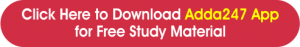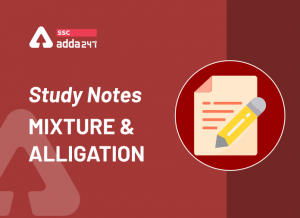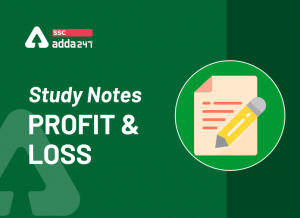Latest SSC jobs   »   Speed, Time And Distance Notes

# Speed, Time And Distance Tricks and Questions with Solutions

## Speed, Time, and Distance

Speed, Time, and Distance: Time, speed, and distance are important concepts of questions asked in Banking, SSC, CAT, and various other competitive exams held every year. While solving the questions, you might get confused about the right formula and tricks to apply to get the answer. In this post, we are going to discuss the important formulas related to speed, time and distance, and relative speed along with examples. The study notes will help you solve the questions related to this topic easily. In this article, we are providing you with the Notes And Questions related to Time, Speed, and Distance.

## Speed, Time, and Distance Formula

a) Distance = Time × Speed
b) When Distance is constant

Time∝1/speed

When Time is constant

Distance ∝ speed

When speed is constant

Distance ∝ Time

c) Average speed = (Total Distance )/(Total Time Taken)

When Distance is equal

Average speed= 2xy/(x + y)
x, y → speeds

### Speed, Time, and Distance Questions

Q1. A car takes half of the time taken by truck to go from Lucknow to Bombay. A truck takes 20 hours to go for the same journey. What is the speed of the truck, if the speed of the car is 120 km/hr.

Q2. Shweta when increasing her speed from 24 km/hr to 30 km/hr she takes one hour less than the usual time to cover a certain distance. What is the distance usually covered by Shweta?

Q3. Kriplani goes to school at 20 km/hr and reaches the school 4 minutes late. Next time, she goes at 25 km/hr and reaches the school 2 minutes earlier than the scheduled time. What is the distance of her school?

Q4. Udai travels half of his journey by train at the speed of 120 km/hr and rests half by car at 80 km/hr. What is the average speed?

Q5. Walking at 4/5 of his normal speed, Dewang is 15 minutes late in reaching his club. What is the usual time taken by him to cover the distance?

### Relative Speed Formula & Questions

(a) When two bodies move in the same direction, Let the speed of two bodies be S_A & S_B.

Relative speed = S_A – S_B

(b) When two bodies are moving in the opposite direction. Let the speed of two bodies be S_A & S_B.

Relative speed = S_A + S_B.

(c) When two bodies move towards each other the time taken by them to meet.

D→ Distance between two bodies.
S_A, S_B→ Speed of two bodies.
T, time is taken to meet each other = D/(S_A+ S_B )

(d) When two bodies are moving in the opposite directions, time is taken to meet.

D→ Distance between the two bodies.
S_A, S_B→ Speed of two bodies
T, time taken = D/(S_A  – S_B )

(e) If two persons A & B, start at the same time from P and Q towards each other and after crossing they take T₁ & T₂ hrs in reaching Q & P

S_A/S_B =√(T_B/T_A )

Q1. The distance between 2 places R and S is 42 km. Anita starts from R with a uniform speed of 4 km/hr towards S and at the same time, Romita starts from S towards R also with some uniform speed. They meet each other after 6 hours. The speed Romita isQ2. The distance between two cities A and B is 330 km. A train starts from A at 8 a.m. and travels towards B at 60 km/hr. Another train starts from B at 9 a.m. and travels towards A at 75 km/hr. At what time do they meet?Q3. A thief Bhagu Ram is spotted by the policeman Pakad Singh from a distance of 200m. Once they see each other they start Running. What is the distance Bhagu Ram, who is running at 5 km/hr would have covered before being caught by Pakad Singh running at 7 km/hr?Q4. A train starts from A to B at 9:00 am and takes 6 hours to travel to B. Another train starts from B to A at 10:00 am and takes 8 hours to travel to A. At what time both train will meet?

Q5. Abhinav leaves Mumbai at 6 am and reaches Bangalore at 10 am Praveen leaves Bangalore at 8 am and reaches Mumbai at 11:30 am. At what time do they cross each other?

## Speed, Time, and Distance: FAQ

Q. What is the relation between Speed, time, and distance?

Ans: You can find the average speed of an object if you know the distance traveled and the time it took. The formula for speed is speed = distance ÷ time.

Q. How do you calculate speed distance and time quickly?

Ans: By using the formula, speed = distance ÷ time you can calculate speed distance, and time.#### SSC CGL Exams Related Links#### Congratulations!General Awareness & Science Capsule PDFIncorrect details? Fill the form again here

General Awareness & Science Capsule PDF

Thank You, Your details have been submitted we will get back to you.
•Mensuration Formula And Questions for 2D...
•Time and Work Notes, Simple Tricks To So...
•Mixture and Alligation, Notes, Methods a...
•Profit and Loss Formula, Concept and Stu...
•Simple Interest Formula, Concept and Stu...
•Pythagoras Theorem: Formula, Proof, Exam...